# Simplified analysis of heat transfer through a finned tube bundle in air cooled condenser-second assessment

Simplified analysis of heat transfer through a finned tube bundle in air cooled condenser-second assessment

Yanán Camaraza-MedinaÁngel M. Rubio-Gonzales Oscar M. Cruz-Fonticiella Osvaldo F. García-Morales Roberto Vizcón- Toledo Ramón Quiza-Sardiñas

Center of Energy Studies and Environmental Technology, Universidad Central de Las Villas, Santa Clara 54440, Cuba

Technical Sciences Faculty, Universidad de Matanzas, Matanzas 44440, Cuba

Corresponding Author Email:
ycamaraza1980@yahoo.com
Page:
365-372
|
DOI:
https://doi.org/10.18280/mmep.050413
24 July 2018
|
Accepted:
3 December 2018
|
Published:
31 December 2018
| Citation

OPEN ACCESS

Abstract:

This paper present a review of mathematical procedures for heat transfer studies in cross flow through finned tube pack. Is given a new model for heat transfer calculations during airflow through finned tubes bank in air cooled condenser systems (ACC). The model was correlated with a total of 783 sets of available experimental data provided by ten authors of recognized prestige in the research area. The air flow bathes a package of finned tubes with an inclination of 45° to 60° with respect to the horizontal line. The studies were performed for ST/SL intervals between 0.4 and 2, air flow velocity of 0.1 to 100 m/s, an ambient temperature of 15 to 43°C, the incident wind velocity over the installation between 0 and 45 km/h, the outer equivalent diameter of the bare tubes between 0.019 and 0.05 m, a fins height between 2.7 and 7.9 mm.The fins thicknesses ranging between 1.3 and 3.5 mm and a number of fins per unit tube length between 315 and 394. The mean deviation found was 6.9% in 85.3% of the correlated experimental data.

Keywords:

airflow, heat transfer coefficient, fins tube bank

1. Introduction

It is well known that in a power cycle the heat exchange is a decisive process in its efficiency, since approximately 90% of the heat extracted from the cycle is done through the condensation system. The waste heat from the steam turbine is released to the atmosphere from the cooling system, which, depending on the environmental conditions, makes this exchange from water circulation systems or direct cooling with the environment [1-3].

In the air cooled condenser (ACC), the refrigerant used is the air. Heat transfer theory states that, in the transverse cross of a solitary tube or tube package is strongly dependent on the thickness of the film that contours the posterior portion of the tube [4-6]. This problem is known in the literature as a paradox of Schlichting, however, in the available and reviewed sources, there are scarcely a dozen works available that have treated the subsequent tubes fluid crossing, according to what is proposed by [5-6].

During the last 50 years many investigations have been carried out to determine the heat transfer and pressure drop over the tube banks. One of the first correlations was established by Grimson  for bare tube banks. A standard reference for the heat-transfer and fraction data of plate-fin heat exchanger surfaces is the book by Kays and London. This book presents j and f versus Reynolds number plots for tube banks, tube-fin heat exchangers, and 52 different plate-fin surface geometries. Shah and Bhatti  summarize important theoretical solutions and correlations for simple geometries that are common in compact heat exchangers. Most of the experimental data have been obtained with air as the test fluid

In this paper, authors present an compacts revision of analysis methods, available in literature, and is provided besides an detail survey over hydrodynamic and heat transfer process in cross fluid over a tube bank, being proposed finally a new method of analysis that includes the effect of the local dry bulb temperature (TTBS), element done not consider in the methods at present known, in spite of his decisive influence over heat transfer coefficient.

2. Materials and Methods

2.1 Transverse fluid crossing of a finned tubes bank

The tubes on a bank are usually arranged in a row or triangle in the direction of flow, as shown in Figure 1. The outside diameter of the tube is taken as the characteristic length. The arrangement of tube bank is characterized by the transverse passage ST, the longitudinal passage SL and the diagonal step SD between the centers of the tubes (see figure 1).

The diagonal step is determined from the following equation:

${{S}_{D}}=\sqrt{S_{L}^{2}+{{\left( \frac{{{S}_{T}}}{2} \right)}^{2}}}$ (1)

In equation (1) (see figure 1 for used notations):

ST is the transverse step, in m

SL is the longitudinal passage, in m

SD is the diagonal step, in m

## 1.png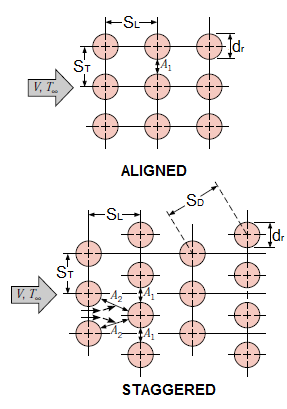Figure 1. Tubes arrangement in a bank

As the fluid enters the bank, the flow area decreases from  ${{A}_{T}}={{S}_{T}}L$ to ${{A}_{T}}=\left( {{S}_{T}}-{{d}_{r}} \right)L$ between the tubes, and as a consequence, the flow velocity increases. In the stepped arrangement the velocity can increase further in the diagonal region if the rows of tubes are very close together. In tube banks the characteristics of the flow are dominated by the maximum velocity Vm which is held within the bank rather than by the approximate velocity V. Therefore, the Reynolds number is defined on the basis of the maximum velocity as:

${{\operatorname{Re}}_{d}}=\frac{\rho {{V}_{m}}d}{\mu }=\frac{{{V}_{m}}d}{\nu }$ (2)

The maximum velocity is determined based on the mass conservation requirement for the incompressible stationary flow. For alignment arrangement, the maximum velocity is in the minimum flow area between the tubes and the mass conservation can be expressed as (see Figure 1):

$\rho {{V}_{0}}{{A}_{1}}=\rho {{V}_{m}}{{A}_{T}}={{V}_{m}}\left( {{S}_{T}}-{{d}_{r}} \right)$ （3）

In equation (3) (see figure 1 for used notations):

• V0 is the entry velocity of the refrigerant to the bundle, in m/s
• dr is the outer diameter of the bare tubes (without fins), in m

Then from equation (3) it follows that the maximum velocity will be as:

${{V}_{Max}}=\frac{{{S}_{T}}}{{{S}_{T}}-d}{{V}_{0}}$  （4）

In the staggered arrangement, fluid approaching through the area A1 (see Figure 1) passes through the area AT and before for area 2AD, as it is wound around the tube in the next row. If it is true that 2AD>AT, the maximum velocity still occurs in AT, between the tubes and consequently, the Vm, in equation (4) can be used for staggered bundles tube.

If 2AD<AT [for $2\left( {{S}_{D}}-d \right)<\left( {{S}_{T}}-d \right)$ ], the maximum velocity will be in the diagonal cross-sections. In this case, maximum velocity is given by:

${{V}_{m}}=\frac{{{S}_{T}}}{2\left( {{S}_{D}}-{{d}_{r}} \right)}{{V}_{0}}$    (5)

The conservation of mass can be expressed as (see Figure 1):

$\rho {{V}_{0}}{{A}_{1}}=\rho {{V}_{m}}\left( 2{{A}_{D}} \right)$    (6)

Or what is the same.

${{V}_{0}}{{S}_{T}}=2{{V}_{m}}\left( {{S}_{D}}-{{d}_{r}} \right)$  (7)

Heat transfer peculiarities in cross flow over a tube bank are very similar to those found in a solitary tube. The variation of thermal transfer around a tube in a bench is determined by the flow pattern, which depends to a large extent on the arrangement of the tubes. Thus in line layout banks, there are two collision points, which results in two thermal transfer peaks. However in banks with staggered arrangement, the process of heat transfer is to some extent similar to that of a solitary tube.

Figure 2 provides a comparison of the quotient arising between the local heat transfer coefficient and the average heat transfer coefficient for a solitary tube and for third row of in-line and staggered arrangements.

## 2.png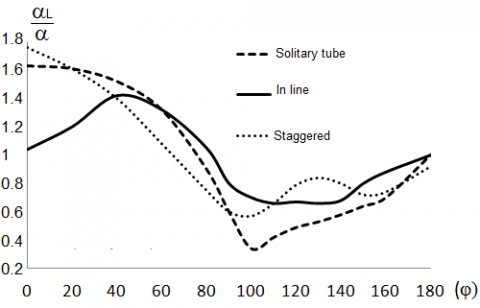Figure 2. Local heat transfer coefficient variation for different arrangements of finned tubes

Figure 2 shows that in in-line arrays the maximum local heat transfer is located at the point ϕ=50º, while the staggered arrangement has two maximum points, one at the point of fluid stagnation ϕ=50º and another in the flow separation. It is expected that the presence of two maximum points will cause the area under the curve to be larger in the staggered arrangements and therefore, taking into account also the low corrosion and deposition properties of the air, make preferable the staggered arrangement for ACC systems, because with this a higher average heat transfer coefficient is achieved. An inspection to ACC manufacturers' catalogs confirms that the staggered arrangement is the most used in all marketed equipment.

2.2 The j and f factors too many configurations

The j factor and fanning f factor are defined rather consistently in the literature by the following equations:

$j=\frac{\alpha {{\left( \Pr \right)}^{{2}/{3}\;}}}{G\cdot Cp}=\frac{\alpha }{G\cdot Cp}\cdot {{\left( \frac{\mu \cdot Cp}{\lambda } \right)}^{{2}/{3}\;}}$   (8)

$\Delta p=4f\frac{L}{{{D}_{h}}}\frac{V_{m}^{2}}{2g}$    (9)

In equation (9) Dh is the equivalent diameter in diagonal cross-sections, and values of Vm is determined by the use of the following relations:

\begin{align} & if\quad 2\left( {{S}_{D}}-{{d}_{r}} \right)>\left( {{S}_{T}}-{{d}_{r}} \right)\quad ;\quad Vm=\frac{{{S}_{T}}}{{{S}_{T}}-{{d}_{r}}}{{V}_{0}} \\ & if\quad 2\left( {{S}_{D}}-{{d}_{r}} \right)\le \left( {{S}_{T}}-{{d}_{r}} \right)\quad ;\quad Vm=\frac{{{S}_{T}}}{2\left( {{S}_{D}}-{{d}_{r}} \right)}{{V}_{0}} \\\end{align}(10)

The correlation given by Giedt  states that the thermal performance of a bare tube bank is given:

$j=\frac{0.376}{{{\operatorname{Re}}^{0.4}}}$       (11)

In-line tube bank, for a 10-row-deep in-line tube bank, the correlation for j is given by :

$j=\frac{0.333}{{{\operatorname{Re}}^{0.4}}}$      (12)

Expressions (11) and (12) are valid for tube diameter from 6 to 50 mm, and Reynolds number range 100 -80 000.

For circular tube-fin arrangement, the basic geometry is shown in figure 3. Experimental heat-transfer and fluid-friction data for three circular finned tube surfaces are given in London et al. [8-10].

## 3.png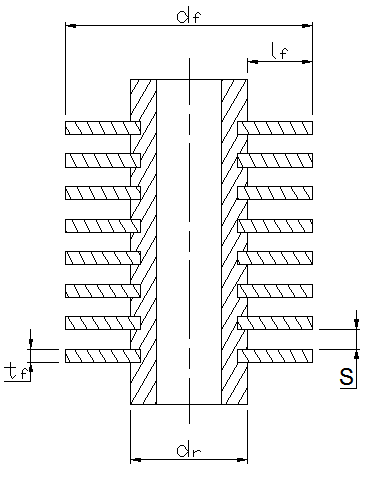Figure 3. Tube-fins details

Briggs and Young Correlations . For low-fin tube banks, the correlation based on experimental heat transfer data is:

$Nu=0.1507{{\operatorname{Re}}^{0.667}}{{\Pr }^{{1}/{3}\;}}{{\left( \frac{S}{{{l}_{F}}} \right)}^{0.164}}{{\left( \frac{S}{{{t}_{F}}} \right)}^{0.075}}$         (13)

where $S=\left[\frac{\left(l-N_{T} t_{F}\right)}{t_{F}}\right]$, with a standar deviation of 3.1%. This is applicable for a circular finned tube with low fin height and high fin density, Re = 1000-20 000, $N_{t} \geq 6$ and equilateral triangular pitch.

For high-fin tube banks the correlation based on experimental heat-transfer data with a standard deviation of 5.1% is:

$Nu=0.1378{{\operatorname{Re}}^{0.718}}{{\Pr }^{{1}/{3}\;}}{{\left( \frac{S}{{{l}_{F}}} \right)}^{0.296}}$   (14)

For all tube banks, the correlation based on regression analysis with a standard deviation of 5.1% is:

\begin{align} & Nu=0.134{{\operatorname{Re}}^{0.681}}{{\Pr }^{{1}/{3}\;}}{{\left( \frac{S}{{{l}_{F}}} \right)}^{0.2}}{{\left( \frac{S}{{{t}_{F}}} \right)}^{0.1134}} \\ & j=0.134{{\operatorname{Re}}^{-0.319}}{{\Pr }^{{1}/{3}\;}}{{\left( \frac{S}{{{l}_{F}}} \right)}^{0.2}}{{\left( \frac{S}{{{t}_{F}}} \right)}^{0.1134}} \\\end{align}.      (15)

Rabas, Eckels and Sabatino Correlations  for tube banks arranged on an equilateral triangular pitch with low-finned tubes is:

$j=0.292{{\operatorname{Re}}^{-m}}{{\Pr }^{{1}/{3}\;}}{{\left( \frac{S}{{{d}_{F}}} \right)}^{1.116}}{{\left( \frac{S}{{{l}_{F}}} \right)}^{0.257}}{{\left( \frac{S}{{{t}_{F}}} \right)}^{-0.66}}\times \times {{\left( \frac{{{d}_{F}}}{{{d}_{r}}} \right)}^{0.47}}{{\left( \frac{{{d}_{F}}}{{{t}_{F}}} \right)}^{0.77}}{{\alpha }_{H}}{{\alpha }_{N}}$    (16)

where $m=0.415-0.0346 \ln \left(\frac{d_{F}}{S}\right)$

In Equation (16) αand αare a temperature-dependent fluid properties correction factor and a row correction factor respectively. The row correction factor has been covered earlier. Rabas and Taborek  recommend the following correlations for αand αN:

${{\alpha }_{H}}={{\left( \frac{{{T}_{b}}+273}{{{T}_{W}}+273} \right)}^{0.25}}\cong {{\left( \frac{\Pr \,\left( {{T}_{b}} \right)}{\Pr \,\left( {{T}_{W}} \right)} \right)}^{0.26}}$  (17)

\begin{align} & for\ {{N}_{T}}\le 8\quad \to \ \ \quad {{\alpha }_{N}}=0,66{{\left( {{N}_{T}} \right)}^{0.21}} \\ & for\ {{N}_{T}}>8\quad \to \ \ \quad {{\alpha }_{N}}=1 \\\end{align} (18)

The fanning friction factor is given by:

$f=3.805{{\operatorname{Re}}^{-0.23}}{{\left( \frac{S}{{{d}_{F}}} \right)}^{0.25}}{{\left( \frac{{{l}_{F}}}{S} \right)}^{0.76}}{{\left( \frac{{{d}_{r}}}{{{d}_{F}}} \right)}^{0.73}}\times {{\left( \frac{{{d}_{F}}}{{{S}_{T}}} \right)}^{0.71}}{{\left( \frac{{{S}_{L}}}{{{S}_{T}}} \right)}^{0.38}}$   (19)

Equation (16) and (19) are applicable for $l_{F} \leq 6.35 m m, \quad 1000<R e \leq 25000 \quad, \quad 4.76<d_{r} \leq$ $31.75 m m, 246 \leq N_{F} \leq 1181$ fins/m $, \quad 15.08<S_{T} \leq$ $111 \mathrm{mm}, 10.32<S_{L} \leq 96.11 \mathrm{mm}, S_{L} \leq S_{T}, d_{F} / S<40$ and $N_{T} \geq 6$

Engineering Sciences Data Unit (ESDU) Correlations  is applicable for $10000<\mathrm{Re} \leq 100000$ and $N_{T} \geq 10$

$j=\frac{0.183{{\alpha }_{H}}{{\alpha }_{N}}{{\Pr }^{0.027}}{{\left( \frac{S}{{{l}_{F}}} \right)}^{0.36}}{{\left( \frac{{{S}_{T}}}{{{d}_{F}}} \right)}^{0.06}}}{{{\operatorname{Re}}^{0.3}}{{\left( \frac{{{d}_{F}}}{{{l}_{F}}} \right)}^{-0.11}}}$  (20)

The fanning friction factor is given by:

$f=\frac{4.71{{\left( \frac{{{l}_{F}}}{S} \right)}^{0.51}}{{\left( \frac{{{S}_{T}}-{{d}_{F}}}{{{S}_{L}}-{{d}_{F}}} \right)}^{0.54}}}{{{\operatorname{Re}}^{0.29}}{{\left( \frac{{{S}_{T}}}{{{d}_{F}}}-1 \right)}^{0.36}}}$  (21)

Gray and Webb Correlations  is applicable for $500<\mathrm{Re} \leq 2.47 \cdot 10^{5}$. To four-row array, j factor is determined by:

${{j}_{4}}=0.14{{\left( \frac{S}{{{d}_{r}}} \right)}^{0.03}}{{\operatorname{Re}}^{-0.33}}{{\left( {{{S}_{T}}}/{{{S}_{L}}}\; \right)}^{-0.5}}$  (22)

Equation (22) correlated within $\pm 10 \%$ in the 89% of availables data. Up to three rows:

$\frac{{{j}_{N}}}{{{j}_{4}}}=0.991\cdot {{\left[ 2.24{{\operatorname{Re}}^{-0.092}}{{\left( \frac{{{N}_{T}}}{4} \right)}^{-0.031}} \right]}^{0.607\cdot \left( 4-{{N}_{T}} \right)}}$ (23)

Equation (22) and (23) are valid for $1 \leq N_{T}, 1.27 \leq S_{T} / d_{r} \leq 2.55,1.4 \leq S_{L} / d_{r} \leq 2.58$ and $0.08 \leq S / d_{r} \leq 0.64$.

2.3 Fluid crossing of a finned tubes bank and his influence on local heat transfer coefficient

A fluid crossing a solitary cylinders (or a package of these) results in flow separation, which is difficult to handle analytically. Therefore, flows of this type should be studied experimentally or numerically. In fact, flow through cylinders and cylinder packs has been studied experimentally by numerous researchers and several empirical correlations have been developed for the determination of heat transfer coefficient [14-16]. The complicated pattern of the flow through a single finned cylinder greatly influences the heat transfer.

Many investigations have been carried out in order to clarify this problem. In figure 4 are given the average values of experimental results reported by [17-18]. This figure provides the variation of the local Nusselt number Nu  depending on the angle $\phi$, on the periphery of a finned cylinder bathed by a cross and perpendicular airflow.

A detailed analysis of figure 4 and the available experimental quantities allows us to establish that in the transversal bathing of a cylinder finned by a turbulent airflow, two basic regions are formed depending on the Reynolds dimensionless number. The first, in interval $4 \cdot 10^{3} \leq R e \leq 10^{5}$, and the second in interval $10^{5}<R e \leq 4 \cdot 10^{5}$.

## 4.png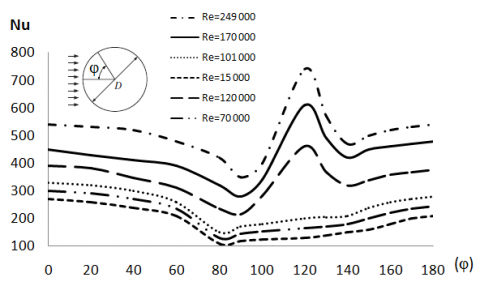Figure 4. Local Nusselt number variation as a function of the angle in air cross flow over finned cylinder

In the first interval, the value of  Nu starts relatively high at the stagnation point $\left(\varphi=0^{\circ}\right)$, reaching at this point its maximum value NuMAX. For $0^{\circ}<\varphi \approx 60^{\circ}$, Nu resents a decreasing tendency very close to a linear function with negative slope $m \approx-1.15$. In interval $60^{0}<\varphi \approx 80^{\circ}$ the downward trend undergoes a sharp change in slope taking now $-5<m \approx-6$. In both cases this decrease is caused by the thickening of the laminar boundary layer.

In the point $\varphi \approx 80^{\circ}$ separation of the laminar flow occurs and therefore it is logical that the number of  Nu reach its minimum value, causing this to also coincide in $\varphi \approx 80^{\circ}$ the presence of a positive inflection point. At this point it is true that $N u \approx\left(N u_{M A X}\right)^{0.84}$. For $80^{0}<\varphi \leq 180^{0}$, Nu present a clear tendency to grow as a result of intense mixing in the region of the separate stream (the wake). This growth follows approximately a positive slope line $m \approx 1,15$ until $\varphi \approx 180^{0}$, where it is true that  $N u \approx 0.9 N u_{M A X}$.

However the second region $10^{5}<\mathrm{Re} \leq 4 \cdot 10^{5}$ its behavior differs from the first. In the first interval, value of Nu  starts relatively high at the point of stagnation $\left(\varphi=0^{\circ}\right)$, reaching at this point a high value. $\left(N u_{0}\right)$ but not maximum as happened in the first region. For $0^{0}<\varphi \approx 90^{\circ} \quad N u$ number present a decreasing tendency very close to a linear function with negative slope $m \approx-1.8$. The point $\varphi \approx 90^{\circ}$ is where the laminar flow separation occurs, and therefore it is logical that the Nusselt number reaches its minimum value and this point is at once an inflection point. At this point it is true that $N u \approx\left(N u_{0}\right)^{0.92}$.

For $90^{0}<\varphi \leq 120^{\circ}$. Nu number present a clear tendency to growth being asymptotically guided by a positive slope whose value varies in the intervals.  12$\approx m \approx 14$. This increase in the local number is due to the transition from laminar to turbulent.  In $\varphi=120^{\circ}$ is where a maximum $\left(N u_{M A X}\right)$  and a second point of inflection are located, what is fulfilled $N u_{M A X}=\left(N u_{0}\right)^{1,05}$.

For $120^{\circ}<\varphi \leq 140^{0}$, Nu number present a pronounced decreasing behavior, not ruled by a single slope, until reaching $\varphi \approx 140^{0}$ , at which point the second minimum of the local Nu number is presented, being fulfilled that $N u=\left(N u_{0}\right)^{0.98}$.

This point is precisely where the separation of the flow occurs in the turbulent flow. For $140^{0}<\varphi \leq 180^{0}$, a new growth of the local number as a result of the intense mixing in the turbulent region of the wake, this growth presents an asymptotic behavior to a linear function with a positive slope that oscillates in the interval 4.1$\approx m \approx 4.8$, is also fulfilled in that, $\varphi=180^{\circ}$ that $N u=(16 / 15) \cdot\left(N u_{0}\right)$.

The discussions given in the previous paragraphs about the local heat transfer coefficients provide insight into the behavior of this at any point in the finned cylinder and for any operant flow regime, however, have little value in heat transfer calculations, since in these, the average heat transfer coefficient over the entire surface is required. The main author provides a detailed discussion of this problem in the reference .

The row variation in the staggered arrangements does not produce significant changes in local heat transfer coefficient. This criterion is confirmed by the authors [8-11], who examined experimentally arrangements with different dimensions and for a fixed value of $\operatorname{Re}=7\cdot {{10}^{4}}$. The results obtained confirm that the local heat transfer coefficient follows approximately a common pattern for all the arrangements examined. In figure 5 are plotted the averages available values.

## 5.pngFigure 5. Local heat transfer coefficient variation for different dimensions in ACC with staggered arrangement

The row variation in aligned arrangements does not produce significant changes in local heat transfer coefficient. This criterion is confirmed by the authors [12-14], who examined experimentally arrangements with different dimensions and for a fixed value of $\mathrm{Re}=3 \cdot 10^{4}$. The results obtained confirm that the local heat transfer coefficient follows approximately a common pattern for all the arrangements examined. In figure 6 are plotted the averages available values.

In any arrangement of tube bank, heat transfer coefficient increases from the first row to the third, and takes a constant value from the latter. In figure 7 and 8 are plotted for staggered and in-line arrangements, respectively, the average experimental values reported by the authors [19-22] for the first three rows of a tube package of an ACC, for $\mathrm{Re}=1.4 \cdot 10^{4}$ and one dimension $(2 x 2) d_{r}$.

## 6.pngFigure 6. Local heat transfer coefficient variation for different dimensions in ACC with aligned arrangement

## 7.png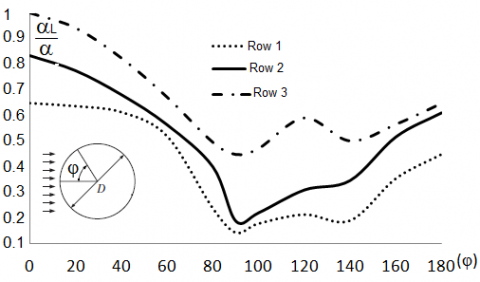Figure 7. Variation of a local heat transfer coefficient in ACC with staggered arrangement

## 8.pngFigure 8. Variation of a local heat transfer coefficient in ACC with aligned arrangement

2.4 Developments of new model and his validation through experimental available data

An analysis of 783 sets of available experimental data reported by a total of 10 authors linked to centers of high prestige [12-15], allowed to develop a dimensionally non-homogeneous expression, which was generated by an integral analysis of residues by cross-methods of breaks at intervals, (Breshnetzov’s method) describing with a mean error of the order $\pm 6.9 \%$ in the 85.3% of available experimental samples. This equation is given by the following expression:

$\alpha =\frac{\left[ 1+Log{{\left( {{{T}_{TBS}}}/{{{V}_{W}}}\; \right)}^{0.015}} \right]\cdot {{\left( {{V}_{m}} \right)}^{0.06}}{{\left( {{t}_{F}}\cdot {{l}_{F}} \right)}^{0.01}}}{\left( 0.15\cdot {{\left( {{S}_{T}}-{{d}_{r}} \right)}^{0.4}} \right)\cdot \left\{ 0.17Ln\left( F \right) \right\}}$         (24)

In Equation (24):

${{T}_{TBS}}$is the dry bulb temperature, in 0C

${{t}_{F}}$is the fins thickness, in mm

${{l}_{F}}$is the fins height, in mm

$F$is the number of fins per linear meter of the finned tube.

${{V}_{W}}$is the incident wind velocity over ACC installation, in km/h

## 9.png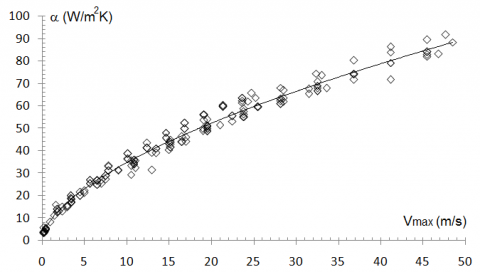Figure 9. Correlation of available experimental samples with equation (24)

Table 1. Comparison between Equation (6) and experimental values available for staggered arrangements in ACC systems

 Source Number of data Fluid (ST /SL) V0 (m/s) TTBS (oC) θ VW (km/h) dr  (m) lF (mm) tF (mm) Fins per meter of tube Deviation percent (%) Kumar et al.  36 Air 0.4 0.1 36 18 35 450 0 25 0.019 2.7 1.3 394 3.2 -0.8 44 Air 1 30 72 20 36 600 0 42 0.0254 6.35 2.5 394 5.1 -1.4 38 Air 1.5 0.9 8 20 32 600 0 36 0.0254 5.1 2.8 394 9.8 3.2 21 Air 2 43 98 18 30 600 0 24 0.0254 7.9 3.0 394 11.1 5.4 VDI 112 Air 0.4 0.5 19 15 42 600 2 28 0.0254 2.7 2.8 315 5.3 -4.7 14 Air 1 0.1 11 15 43 450 4 40 0.019 5.1 2.5 115 8.9 3.7 78 Air 1.5 0.9 20 18 40 600 0 32 0.0254 5.1 2.8 115 10.2 4.4 44 Air 2 0.4 13 18 32 600 0 40 0.0254 6.35 2.8 115 7.7 1.6 O’Donovan et al. (2015) 14 Air 1 0.1 8 17 35 600 0 35 0.0254 4.7 2.8 254 8.3 0.7 18 Air 1.5 51 100 17 30 600 0 35 0.0254 6.35 1.3 354 -2.4 -9.2 11 Air 2 42 98 15 38 600 0 35 0.019 7.9 2.5 254 11.3 6.1 Morthensen et al. (2013) 40 Air 0.4 41 80 18 35 600 0 42 0.0254 4.7 2.8 354 7.5 4.3 31 Air 1 0.1 10 18 35 600 0 42 0.035 7.9 3.2 354 8.2 1.9 27 Air 1.5 0.1 10 18 35 450 0 42 0.019 7.9 3.5 354 -0.5 -4.8 33 Air 2 0.1 9 18 35 600 0 42 0.0254 2.7 2.3 354 10.8 7.2 Heyns- Kröger (2015) 7 Air 1.5 1.1 9 26 35 600 0 45 0.0254 4.7 2.5 354 9.2 -5.4 4 Air 2 2.3 5 28 32 600 0 40 0.019 4.7 2.3 354 11.8 9.1

Table 2. Comparison between equation (6) and experimental values available for staggered arrangements in ACC systems. (cont.)

 Source Number of data Fluid (ST /SL) V0 (m/s) TTBS (oC) θ VW (km/h) dr  (m) lF (mm) tF (mm) Fins per meter of tube Deviation percent (%) Owen-Kröger  (2013) 18 Air 0.4 0.3 7 16 34 600 0 28 0.0254 4.7 2.5 194 12.3 6.1 13 Air 1 1.1 8 15 31 600 0 28 0.0254 3.8 2.8 154 11.2 7.4 19 Air 1.5 23 60 15 40 600 0 30 0.0254 6.35 3 194 5.1 4.4 21 Air 2 1.8 5 24 36 450 0 35 0.035 6.35 2.8 354 4.5 -6.3 Ingadottir Bara (2015) 18 Air 1 14 67 21 27 600 6 24 0.0254 6.35 2.3 354 6.1 -4.8 19 Air 1.5 1.2 9 22 29 600 7 32 0.035 6.35 2.8 315 5.3 2.2 21 Air 2 1.8 8.1 24 30 600 4 44 0.035 6.35 2.5 354 5.1 -4.2 N. H. Khandy (2015) 28 Air 1 1.2 6 18 30 450 0 35 0.0254 7.9 2.5 354 6.1 1.9 33 Air 1.5 18 50 18 30 450 0 35 0.035 7.9 2.8 354 9.7 -3.2 21 Air 2 1.1 7.1 18 30 450 0 35 0.05 4.7 3 354 -3.2 -8.1 Resume 783 0.4 2 0.1 100 15 43 450 600 0 45 0.019 0.05 2.7 7.9 1.3 3.5 115 394 7.3 1.1

Expression (24) is valid for $15 \leq T_{T B S} \leq 43^{\circ} C$, an refrigerant velocity between 0.1 and 100 m/s, ratios $0.4<S_{T} / S_{L}<2$, external diameters of bare tubes $0.019<d_{r}<0.05 \mathrm{m}$, a wind velocity incident over installation between 0 and 45 km/h, a fins height between 2.7 and 7.9 mm, a fins thickness between 1.3 and 3.5 mm and a number of fins per unit of pipe length between 115 and 394. Table 1 provides a summary of the main parameters of the experimental quantities available and the mean deviation values found in each case. Figure 7 gives the correlation of available experimental samples with equation (6).

3. Conclusions

In the study presented, a new and improved model has been developed to estimate the heat transfer coefficient, in airflow transversal cross to a package of finned tubes used in an ACC system. In the same, it could be verified that the expression obtained (24) is valid to $15\le {{T}_{TBS}}\le 43\,{}^{0}C$, for a refrigerant velocity between 0.1 and 100 m/s, ratios $0.4<{{{S}_{T}}}/{{{S}_{L}}}\;<2$, external diameters of bare tubes$0.019<{{d}_{r}}<0.05\ m$,a wind velocity incident over installation between 0 and 45 km/h, a fins height between 2.7 and 7.9 mm, a fins thickness between 1.3 and 3.5 mm and a number of fins per unit of pipe length between 115 and 394.

783 sets of available experimental data found a mean error of the order $\pm 6.9%$ for 85.3% of the correlated experimental samples. The correlation of available experimental data with expression (24) was shown in Table 1, and it can be verified that the proposed new model yields satisfactory results in the field of action for which it was developed, reducing in more than half error rates obtained with the use of the Zukauskas or Griminson equations in ACC systems [23-25].

A dimensionally non-homogeneous expression was obtained that responds to the following equation:

$\alpha =\frac{\left[ 1+Log{{\left( {{{T}_{TBS}}}/{{{V}_{W}}}\; \right)}^{0.015}} \right]\cdot {{\left( {{V}_{m}} \right)}^{0.06}}{{\left( {{t}_{F}}\cdot {{l}_{F}} \right)}^{0.01}}}{\left( 0.15\cdot {{\left( {{S}_{T}}-{{d}_{r}} \right)}^{0.4}} \right)\cdot \left\{ 0.17Ln\left( F \right) \right\}}$.

Acknowledgment

This work was supported by Doctoral Research Program of Universidad Central “Marta Abreu” de las Villas, Cuba.

Nomenclature
 F number of fins per linear meter of tube lF Fins height, mm tF Fins thickness, mm TTBS Dry bulb temperature, oC Vm Maximum velocity in the minimum flow area, m.s-1 VW Wind velocity over ACC installation , m.s-1 de Inside equivalent diameter of tubes, m ST Transverse step, m SL Longitudinal passage, m SD Diagonal step, m dr external diameter of the tubes with fins, m V0 Velocity of refrigerant entry to the bundle, m.s-1 Greek symbols aL Local heat transfer coefficient. kg.m-2.s-3.K-1 α Mean heat transfer coefficient. kg.m-2.s-3.K-1 φ Angle in air cross flow over finned cylinder, degrees θ ACC tubes inclination respect to horizontal line Subscripts Eq. Equation
References

 Dorao CA, Fernandhino M. (2017). Dominant dimensionless groups controlling heat transfer coefficient during flow condensation inside pipes. International Journal of Heat and Mass Transfer 112(2017): 465-479. https://doi.org/10.1016/j.ijheatmasstransfer.2017.04.104

 Boyko LD, Kruzhilin GN. (1967). Heat transfer and hydraulic resistance during condensation of steam in a horizontal tube and in a bundle of tubes. International Journal of Heat and Mass Transfer 10(3): 361-373. https://doi.org/10.1016/0017-9310(67)90152-4

 Kim SM, Mudawar I. (2013). Universal approach to predicting heat transfer coefficient for condensing mini/micro-channel flow. International Journal of Heat and Mass Transfer 56(1–2): 238–250. http://dx.doi.org/10.1016/j.ijheatmasstransfer.2012.09.032.

 Camaraza Y. (2017). Introducción a la termotransferencia. Ed. Universitaria, La Habana, Cuba. ISBN: 978-959-16-3286-9.

 Medina YC, Khandy NH, Fonticiella OMC, Morales OFG. (2017). Abstract of heat transfer coefficient modelation in single-phase systems inside pipes. Mathematical Modelling of Engineering Problems 4(3): 126-131. https://doi.org/10.18280/mmep.040303

 Rifert VG, Sereda VV. (2015). Condensation inside smooth horizontal tubes: Part 1. Survey of the methods of heat-exchange prediction. Thermal Science 19(5): 1769-1789. https://doi.org/10.2298/TSCI140522036R.

 Grimmison ED. (1937). Correlation and utilization of new data on flow resistance and heat transfer for cross flow of gases over tube banks, Truns. ASME 59: 583-594.

 Shah RK, Bhatti MS. (1988) Assessment of correlations for single-phase heat exchangers. In Two-Phase Flow Heat Exchangers-Thermal-Hydraulic Fundamentals and Design Kakac S, Bergles A E, and F. E. Oliveira, ed. Kluwer Academic Publishers, London, pp. 81-122.

 Giedt WH. (1957). Principles of engineering heat transfer, ed. Van Nostrand, New York, pp. 136-137.

 Briggs DE, Young EH. (1963). Convection heat transfer and pressure drop of air flowing across triangular pitch banks of finned tubes. Chem. Eng. Prog. Symp. Ser. 59: 1-10.

 Robinson KK, Briggs DE. (1966). Pressure drop of air flowing across triangular pitch banks of finned tubes. Chem. Eng. Prog. Symp. Ser. 62: 177-184.

 Rabas TJ, Taborek J. (1987). Survey of turbulent forced convection heat transfer and pressure drop characteristics of low finned tube banks in cross flow. Heat Transfer Engineering 8: 49-61.

 Gray DL, Webb RL. (1986). Heat transfer and friction correlations for plate fin and tube heat exchangers having plain fins. Heat Transfer 1986, Proc. Eighth Int. Heat Transfer Conference 6: 2745-2750.

 Cttani L, Bozzoli F, Raineri S. (2017). Experimental study of the transitional flow regime in coiled tubes by the estimation of local convective heat transfer coefficient. International Journal of Heat and Mass Transfer 108(2017): 825-836. https://doi.org/10.1016/j.ijheatmasstransfer.2017.05.003

 Ghim G, Lee J. (2017). Condensation heat transfer of low GWP ORC working fluids in a horizontal smooth tube. International Journal of Heat and Mass Transfer 104(2017): 718-728. https://doi.org/10.1016/j.ijheatmasstransfer.2016.08.090

 Camaraza-Medina Y, Cruz-Fonticiella OM, García-Morales OF. (2018). Predicción de la presión de salida de una turbina acoplada a un condensador de vapor refrigerado por aire. Centro Azúcar 45(1): 50-61.

 Pourmahmoud N, Abbaszadeh M, Rashidzadeh M. (2016). Numerical simulation of effect of shell heat transfer on the vortex tube performance. International Journal of Heat and Technology 34(2): 293-301. http://doi.org/10.18280/ijht.340220000

 Zhang ZY, Yang JG. (2015). The effect of face-air velocity distribution on heat transfer performance of air-cooled condensers. International Journal of Heat and Technology 33(1): 55-62. http://doi.org/10.18280/ijht.330108

 Kumar A, Joshi JB, Nayak AK, Vijayan PK. (2016). 3D CFD simulations of air cooled condenser-II: Natural draft around a single finned tube kept in a small chimney. International Journal of Heat and Mass Transfer 92(2016): 507-522. https://doi.org/10.1016/j.ijheatmasstransfer.2015.07.136

 Alam MS, Islam T, Uddin MJ. (2016). Mathematical modelling for heat transfer of a micropolar fluid along a permeable stretching/shrinking wedge with heat generation/absorption. Mathematical Modelling of Engineering Problems 3(1): 1-9. https://doi.org/10.18280/mmep.030101

 Zhang W, Du X, Yang L, Yang Y. (2016). Research on performance of finned tube bundles of indirect air-cooled heat exchangers. Mathematical Modelling of Engineering Problems 3(1): 47-51. https://doi.org/10.18280/mmep.030108

 Camaraza-Medina Y, Khandy NH, Carlson KM, Cruz-Fonticiella OM, García-Morales OF, Reyes-Cabrera D. (2018). Evaluation of condensation heat transfer in air-cooled condenser by dominant flow criteria. Mathematical Modelling of Engineering Problems 5(2): 76-82. https://doi.org /10.18280/mmep.050204

 Medina YC, Fonticiella OMC, Morales OFG. (2017). Design and modelation of piping systems by means of use friction factor in the transition turbulent zone. Mathematical Modelling of Engineering Problems 4(4): 162-167. https://doi.org /10.18280/mmep.040404

 Medina YC, Khandy NH, Carlson KM, Fonticiella OMC, Morales OFG. (2018). Mathematical modeling of two-phase media heat transfer coefficient in air cooled condenser system. International Journal of Heat and Technology 36(1): 319-324. https://doi.org/10.18280/ijht.360142

 Camaraza-Medina Y, Hernandez-Guerrero A, Luviano-Ortiz JL, Mortensen-Carlson K, Cruz-Fonticiella OM, García-Morales O.F. (2018). New model for heat transfer calculation during film condensation inside pipes. International Journal of Heat and Mass Transfer 128(2019): 344-353. https://doi.org/10.1016/j.ijheatmasstransfer.2018.09.012